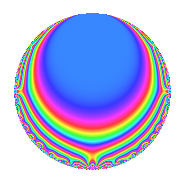# Properties

 Label 600.1.bj.aLevel 600 Weight 1 Character orbit 600.bj Analytic conductor 0.299 Analytic rank 0 Dimension 4 Projective image $$D_{5}$$ CM disc. -24 Inner twists 4

# Related objects

## Newspace parameters

 Level: $$N$$ = $$600 = 2^{3} \cdot 3 \cdot 5^{2}$$ Weight: $$k$$ = $$1$$ Character orbit: $$[\chi]$$ = 600.bj (of order $$10$$ and degree $$4$$)

## Newform invariants

 Self dual: No Analytic conductor: $$0.29943900758$$ Analytic rank: $$0$$ Dimension: $$4$$ Coefficient field: $$\Q(\zeta_{10})$$ Coefficient ring: $$\Z[a_1, a_2]$$ Coefficient ring index: $$1$$ Projective image $$D_{5}$$ Projective field Galois closure of 5.1.225000000.2

## $q$-expansion

The $$q$$-expansion and trace form are shown below.

 $$f(q)$$ $$=$$ $$q -\zeta_{10} q^{2} + \zeta_{10}^{2} q^{3} + \zeta_{10}^{2} q^{4} -\zeta_{10} q^{5} -\zeta_{10}^{3} q^{6} + ( \zeta_{10}^{2} - \zeta_{10}^{3} ) q^{7} -\zeta_{10}^{3} q^{8} + \zeta_{10}^{4} q^{9} +O(q^{10})$$ $$q -\zeta_{10} q^{2} + \zeta_{10}^{2} q^{3} + \zeta_{10}^{2} q^{4} -\zeta_{10} q^{5} -\zeta_{10}^{3} q^{6} + ( \zeta_{10}^{2} - \zeta_{10}^{3} ) q^{7} -\zeta_{10}^{3} q^{8} + \zeta_{10}^{4} q^{9} + \zeta_{10}^{2} q^{10} + ( 1 + \zeta_{10}^{2} ) q^{11} + \zeta_{10}^{4} q^{12} + ( -\zeta_{10}^{3} + \zeta_{10}^{4} ) q^{14} -\zeta_{10}^{3} q^{15} + \zeta_{10}^{4} q^{16} + q^{18} -\zeta_{10}^{3} q^{20} + ( 1 + \zeta_{10}^{4} ) q^{21} + ( -\zeta_{10} - \zeta_{10}^{3} ) q^{22} + q^{24} + \zeta_{10}^{2} q^{25} -\zeta_{10} q^{27} + ( 1 + \zeta_{10}^{4} ) q^{28} + 2 \zeta_{10}^{2} q^{29} + \zeta_{10}^{4} q^{30} + ( 1 - \zeta_{10} ) q^{31} + q^{32} + ( \zeta_{10}^{2} + \zeta_{10}^{4} ) q^{33} + ( -\zeta_{10}^{3} + \zeta_{10}^{4} ) q^{35} -\zeta_{10} q^{36} + \zeta_{10}^{4} q^{40} + ( 1 - \zeta_{10} ) q^{42} + ( \zeta_{10}^{2} + \zeta_{10}^{4} ) q^{44} + q^{45} -\zeta_{10} q^{48} + ( 1 - \zeta_{10} + \zeta_{10}^{4} ) q^{49} -\zeta_{10}^{3} q^{50} + ( -\zeta_{10} - \zeta_{10}^{3} ) q^{53} + \zeta_{10}^{2} q^{54} + ( -\zeta_{10} - \zeta_{10}^{3} ) q^{55} + ( 1 - \zeta_{10} ) q^{56} -2 \zeta_{10}^{3} q^{58} + ( 1 - \zeta_{10}^{3} ) q^{59} + q^{60} + ( -\zeta_{10} + \zeta_{10}^{2} ) q^{62} + ( -\zeta_{10} + \zeta_{10}^{2} ) q^{63} -\zeta_{10} q^{64} + ( 1 - \zeta_{10}^{3} ) q^{66} + ( 1 + \zeta_{10}^{4} ) q^{70} + \zeta_{10}^{2} q^{72} -2 \zeta_{10} q^{73} + \zeta_{10}^{4} q^{75} + ( 1 + \zeta_{10}^{2} - \zeta_{10}^{3} + \zeta_{10}^{4} ) q^{77} + ( -\zeta_{10} - \zeta_{10}^{3} ) q^{79} + q^{80} -\zeta_{10}^{3} q^{81} + ( 1 - \zeta_{10} ) q^{83} + ( -\zeta_{10} + \zeta_{10}^{2} ) q^{84} + 2 \zeta_{10}^{4} q^{87} + ( 1 - \zeta_{10}^{3} ) q^{88} -\zeta_{10} q^{90} + ( \zeta_{10}^{2} - \zeta_{10}^{3} ) q^{93} + \zeta_{10}^{2} q^{96} + ( 1 + \zeta_{10}^{4} ) q^{97} + ( 1 - \zeta_{10} + \zeta_{10}^{2} ) q^{98} + ( -\zeta_{10} + \zeta_{10}^{4} ) q^{99} +O(q^{100})$$ $$\operatorname{Tr}(f)(q)$$ $$=$$ $$4q - q^{2} - q^{3} - q^{4} - q^{5} - q^{6} - 2q^{7} - q^{8} - q^{9} + O(q^{10})$$ $$4q - q^{2} - q^{3} - q^{4} - q^{5} - q^{6} - 2q^{7} - q^{8} - q^{9} - q^{10} + 3q^{11} - q^{12} - 2q^{14} - q^{15} - q^{16} + 4q^{18} - q^{20} + 3q^{21} - 2q^{22} + 4q^{24} - q^{25} - q^{27} + 3q^{28} - 2q^{29} - q^{30} + 3q^{31} + 4q^{32} - 2q^{33} - 2q^{35} - q^{36} - q^{40} + 3q^{42} - 2q^{44} + 4q^{45} - q^{48} + 2q^{49} - q^{50} - 2q^{53} - q^{54} - 2q^{55} + 3q^{56} - 2q^{58} + 3q^{59} + 4q^{60} - 2q^{62} - 2q^{63} - q^{64} + 3q^{66} + 3q^{70} - q^{72} - 2q^{73} - q^{75} + q^{77} - 2q^{79} + 4q^{80} - q^{81} + 3q^{83} - 2q^{84} - 2q^{87} + 3q^{88} - q^{90} - 2q^{93} - q^{96} + 3q^{97} + 2q^{98} - 2q^{99} + O(q^{100})$$

## Character Values

We give the values of $$\chi$$ on generators for $$\left(\mathbb{Z}/600\mathbb{Z}\right)^\times$$.

 $$n$$ $$151$$ $$301$$ $$401$$ $$577$$ $$\chi(n)$$ $$1$$ $$-1$$ $$-1$$ $$\zeta_{10}^{2}$$

## Embeddings

For each embedding $$\iota_m$$ of the coefficient field, the values $$\iota_m(a_n)$$ are shown below.

For more information on an embedded modular form you can click on its label.

Label $$\iota_m(\nu)$$ $$a_{2}$$ $$a_{3}$$ $$a_{4}$$ $$a_{5}$$ $$a_{6}$$ $$a_{7}$$ $$a_{8}$$ $$a_{9}$$ $$a_{10}$$
221.1
 −0.309017 + 0.951057i 0.809017 + 0.587785i 0.809017 − 0.587785i −0.309017 − 0.951057i
0.309017 0.951057i −0.809017 0.587785i −0.809017 0.587785i 0.309017 0.951057i −0.809017 + 0.587785i −1.61803 −0.809017 + 0.587785i 0.309017 + 0.951057i −0.809017 0.587785i
341.1 −0.809017 0.587785i 0.309017 + 0.951057i 0.309017 + 0.951057i −0.809017 0.587785i 0.309017 0.951057i 0.618034 0.309017 0.951057i −0.809017 + 0.587785i 0.309017 + 0.951057i
461.1 −0.809017 + 0.587785i 0.309017 0.951057i 0.309017 0.951057i −0.809017 + 0.587785i 0.309017 + 0.951057i 0.618034 0.309017 + 0.951057i −0.809017 0.587785i 0.309017 0.951057i
581.1 0.309017 + 0.951057i −0.809017 + 0.587785i −0.809017 + 0.587785i 0.309017 + 0.951057i −0.809017 0.587785i −1.61803 −0.809017 0.587785i 0.309017 0.951057i −0.809017 + 0.587785i
 $$n$$: e.g. 2-40 or 990-1000 Significant digits: Format: Complex embeddings Normalized embeddings Satake parameters Satake angles

## Inner twists

Char. orbit Parity Mult. Self Twist Proved
1.a Even 1 trivial yes
24.h Odd 1 CM by $$\Q(\sqrt{-6})$$ yes
25.d Even 1 yes
600.bj Odd 1 yes

## Hecke kernels

This newform can be constructed as the kernel of the linear operator $$T_{11}^{4} - 3 T_{11}^{3} + 4 T_{11}^{2} - 2 T_{11} + 1$$ acting on $$S_{1}^{\mathrm{new}}(600, [\chi])$$.